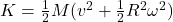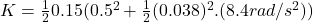## What is the total kinetic energy of a 0.15 kg hockey puck sliding at 0.5 m/s and rotating about its center at 8.4 rad/s

Question

What is the total kinetic energy of a 0.15 kg hockey puck sliding at 0.5 m/s and rotating about its center at 8.4 rad/s

in progress 0
7 months 2021-07-12T12:01:17+00:00 1 Answers 6 views 0

## Answers ( )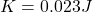Explanation:

From the question we are told that:

Mass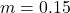Velocity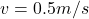Angular Velocity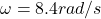Generally the equation for Kinetic Energy is mathematically given by